# ISEE Upper Level Quantitative : How to find the length of the side of a right triangle

## Example Questions

### Example Question #41 : Isee Upper Level (Grades 9 12) Quantitative Reasoning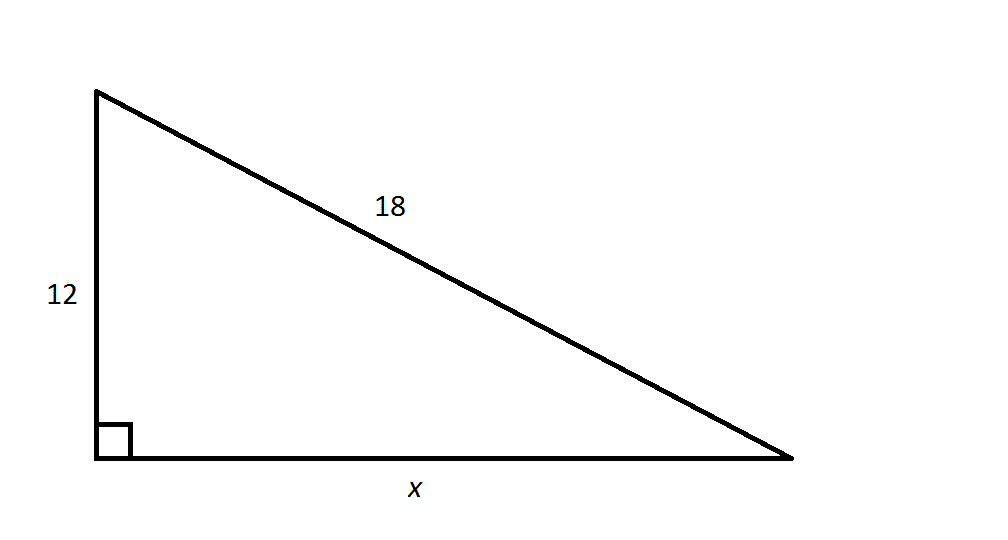Refer to the above right triangle. Which of the following is equal to?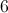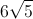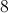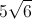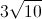Explanation:

By the Pythagorean Theorem,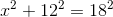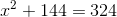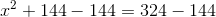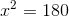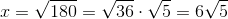### Example Question #2 : How To Find The Length Of The Side Of A Right Triangle

Given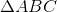with right angle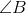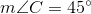Which is the greater quantity?

(a)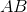(b)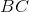(b) is greater.

(a) is greater.

(a) and (b) are equal.

It is impossible to tell from the information given.

(a) and (b) are equal.

Explanation: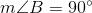The sum of the measures of the angles of a triangle is, so: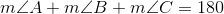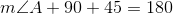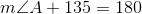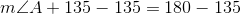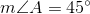This is a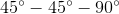triangle, so its legs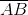and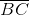are congruent. The quantities are equal.

### Example Question #3 : How To Find The Length Of The Side Of A Right Triangle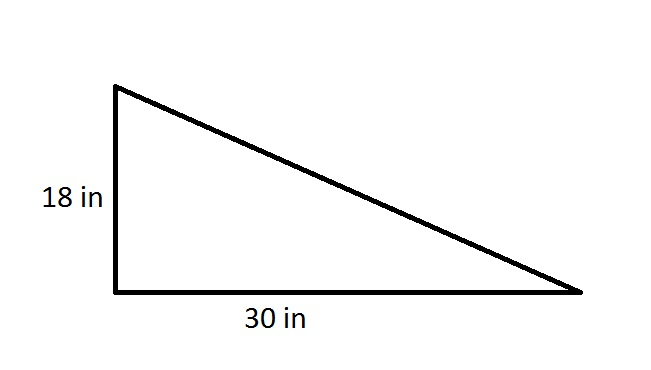Give the length of one leg of an isosceles right triangle whose area is the same as the right triangle in the above diagram.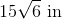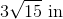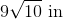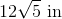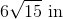Explanation:

The area of a triangle is half the product of its height and its base; in a right triangle, the legs, being perpendicular, can serve as these quantites.

The triangle in the diagram has area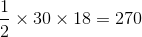square inches.

An isosceles right triangle has two legs of the same length, which we will call. The area of that triangle, which is the same as that of the one in the diagram, is therefore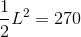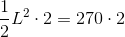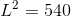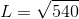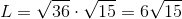inches.

### Example Question #4 : How To Find The Length Of The Side Of A Right Triangle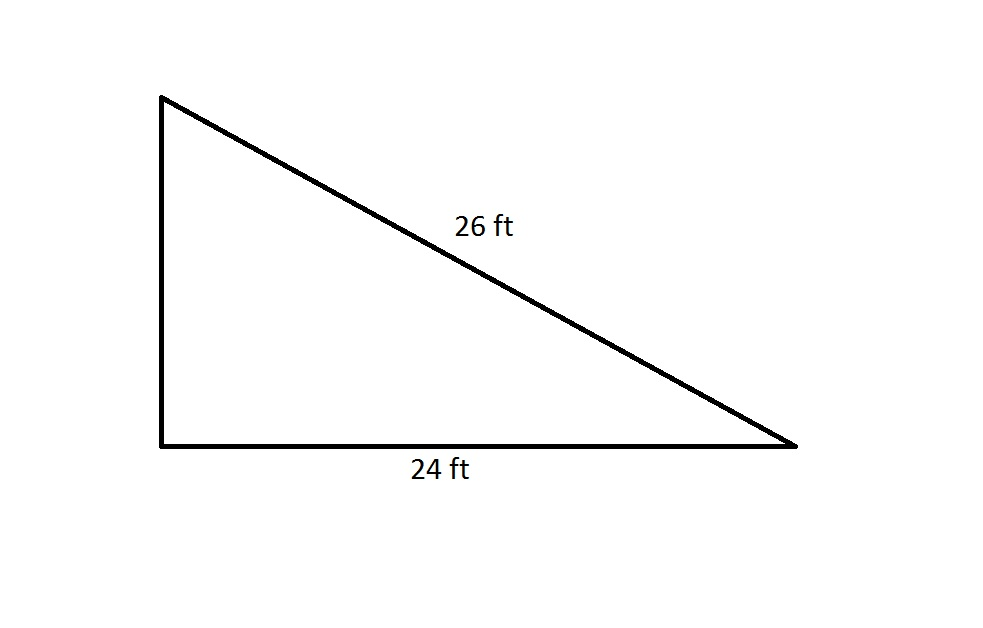The perimeter of a regular octagon is 20% greater than that of the above right triangle. Which is the greater quantity?

(A) The length of one side of the octagon

(B) 3 yards

(B) is greater

It is impossible to determine which is greater from the information given

(A) is greater

(A) and (B) are equal

(A) and (B) are equal

Explanation:

By the Pythagorean Theorem, the shorter leg has length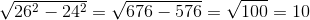feet.

The perimeter of the right triangle is therefore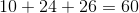feet.

The octagon has perimeter 20% greater than this, or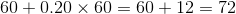feet.

A regular octagon has eight sides of equal length, so each side of this octagon has length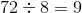feet, which is equal to 3 yards. This makes the quantities equal.

### Example Question #5 : How To Find The Length Of The Side Of A Right TriangleThe area of a square is equal to that of the above right triangle. Which is the greater quantity?

(A) The sidelength of the square

(B) 4 yards

(A) and (B) are equal

It is impossible to determine which is greater from the information given

(B) is greater

(A) is greater

(B) is greater

Explanation:

By the Pythagorean Theorem, the shorter leg has lengthfeet.

The area of a triangle is equal to half the product of its base and height; for a right triangle, the legs can serve as these. The area of the above right triangle is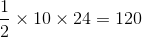square feet.

The sidelength is the square root of this;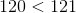, so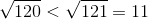. Therefore each sidelength of the square is just under 11 feet. 4 yards is 12 feet, so (B) is greater.

### Example Question #51 : Isee Upper Level (Grades 9 12) Quantitative Reasoning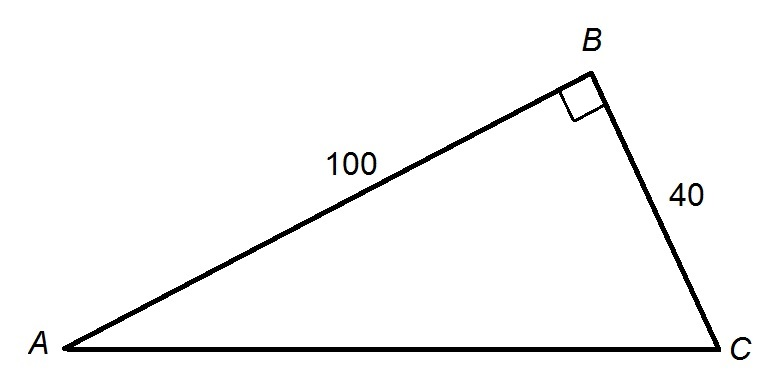Figure NOT drawn to scale.

Refer to the above triangle. Which is the greater quantity?

(a)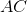(b) 108

It is impossible to determine which is greater from the information given

(b) is the greater quantity

(a) is the greater quantity

(a) and (b) are equal

(b) is the greater quantity

Explanation:

We can compare these numbers by comparing their squares.

By the Pythagorean Theorem,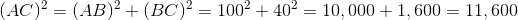Also,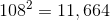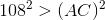, so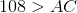.

### Example Question #7 : How To Find The Length Of The Side Of A Right Triangle

Consider a triangle,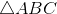, in which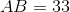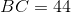, and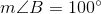. Which is the greater quantity?

(a) 55

(b)(b) is the greater quantity

It is impossible to determine which is greater from the information given

(a) and (b) are equal

(a) is the greater quantity

(b) is the greater quantity

Explanation:

Suppose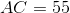.

By the Converse of the Pythagorean Theorem, a triangle is right if and only if the sum of the squares of the lengths of the smallest two sides is equal to the square of the longest side. Compare the quantities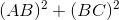and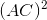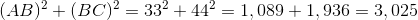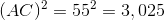Therefore, if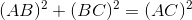, sois right, with the right angle opposite longest side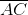. Thus,is right and has degree measure 90.

However,has degree measure greater than 90, so, as a consequence of the Converse of the Pythagorean Theorem and the SAS Inequality Theorem, it holds that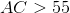.